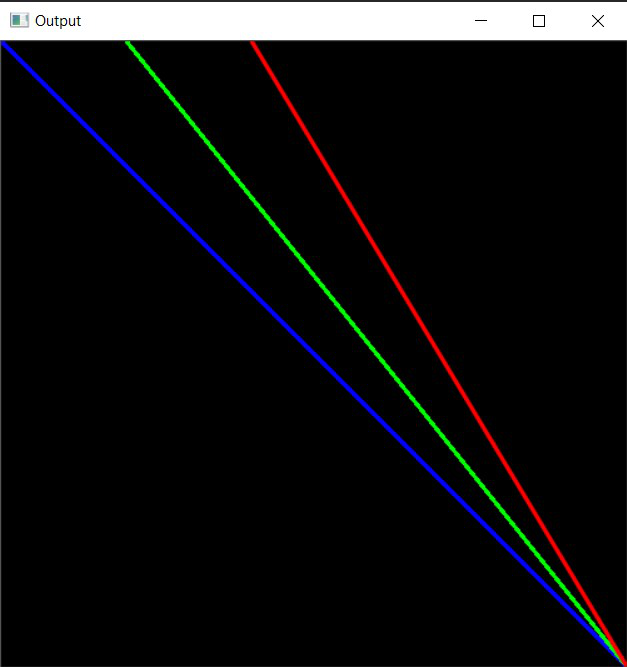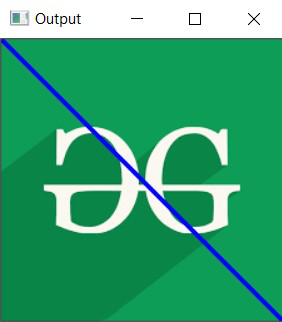Open in App
Not now

# Draw a line using OpenCV in C++

• Last Updated : 27 Jan, 2021

In this article, we will discuss how to draw a line using OpenCV in C++. The idea is to use the line() function from OpenCV C++ library.

Syntax:

line(img, pt1, pt2, color, thickness, lineType, shift)

Parameters:

• img: This is the image file.
• start: Start point of the line segment. The first point out of two ends of a line segment. It is a tuple of two coordinates (x-coordinate, y-coordinate)).
• end: Endpoint of the line segment. The second point out of two ends of a line segment. It is a tuple of two coordinates (x-coordinate, y-coordinate)).
• color: Color of the line to be drawn. It is a tuple representing 3 colors (B, G, R) i.e.. (Blue, Green, Red).
• thickness: Thickness of the line drawn.
• lineType: Type of the line. There are 3 types of line:
• LINE_4: Line was drawn using 4 connected Bresenham algorithm.
• LINE_8: Line drawn using 8 connected Bresenham algorithm.
• LINE_AA: It draws Antialiased lines formed by using the Gaussian filter.
• nshift: It is the Number of fractional bits in the point coordinates.

Return Value: It returns an image.

Program 1:

Below is the program shows how to draw all types of lines over a self-formed background image:

## C++

 `// C++ program for the above approach``#include ``#include `` ` `// Library to include for``// drawing shapes``#include ``#include ``using` `namespace` `cv;``using` `namespace` `std;`` ` `// Driver Code``int` `main(``int` `argc, ``char``** argv)``{``    ``// Create a blank image of size``    ``// (500 x 500) with black``    ``// background (B, G, R) : (0, 0, 0)``    ``Mat image(500, 500, CV_8UC3,``              ``Scalar(0, 0, 0));`` ` `    ``// Check if the image is created``    ``// successfully``    ``if` `(!image.data) {``        ``cout << ``"Could not open or find"``             ``<< ``" the image"``;`` ` `        ``return` `0;``    ``}`` ` `    ``Point p1(0, 0), p2(100, 0);``    ``Point p3(200, 0), p4(500, 500);``    ``int` `thickness = 2;`` ` `    ``// Line drawn using 8 connected``    ``// Bresenham algorithm``    ``line(image, p1, p4, Scalar(255, 0, 0),``         ``thickness, LINE_8);`` ` `    ``// Line drawn using 4 connected``    ``// Bresenham algorithm``    ``line(image, p2, p4, Scalar(0, 255, 0),``         ``thickness, LINE_4);`` ` `    ``// Antialiased line``    ``line(image, p3, p4, Scalar(0, 0, 255),``         ``thickness, LINE_AA);`` ` `    ``// Show our image inside window``    ``imshow(``"Output"``, image);``    ``waitKey(0);`` ` `    ``return` `0;``}`

Output:Program 2:

Below is the program to shows how to draw a line over a loaded image:

## C++

 `// C++ program for the above approach``#include ``#include `` ` `// Library to include for``// drawing shapes``#include ``#include ``using` `namespace` `cv;``using` `namespace` `std;`` ` `// Driver code``int` `main(``int` `argc, ``char``** argv)``{``    ``// Path of the image file``    ``Mat image = imread(``        ``"C:/Users/harsh/Downloads/geeks.png"``,``        ``IMREAD_COLOR);`` ` `    ``// Check if the image is loaded``    ``// successfully``    ``if` `(!image.data) {``        ``std::cout << ``"Could not open or "``                     ``"find the image"``;``        ``return` `0;``    ``}`` ` `    ``Point p1(0, 0), p2(250, 250);``    ``int` `thickness = 2;`` ` `    ``// Line drawn using 8 connected``    ``// Bresenham algorithm``    ``line(image, p1, p2, Scalar(255, 0, 0),``         ``thickness, LINE_8);`` ` `    ``// Show our image inside window``    ``imshow(``"Output"``, image);``    ``waitKey(0);`` ` `    ``return` `0;``}`

Output:My Personal Notes arrow_drop_up